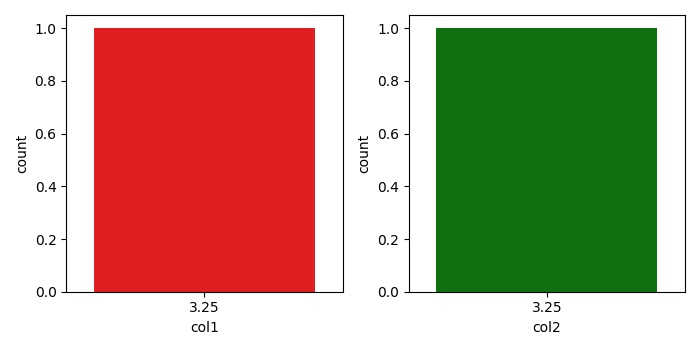# How to limit the number of groups shown in a Seaborn countplot using Matplotlib?

To limit the number of groups shown in a Seaborn countplot, we can use a variable group_count, used in countplot() method arguments.

## Steps

• Create a figure and two sets of subplots.

• Create a data frame using Pandas, with two keys.

• Initalize a variable group_count to limit the group count in countplot() method.

• Use countplot() method to show the counts of observations in each categorical bin using bars.

## Example

import pandas as pd
import numpy as np
import seaborn as sns
from matplotlib import pyplot as plt
plt.rcParams["figure.figsize"] = [7.00, 3.50]
plt.rcParams["figure.autolayout"] = True
f, axes = plt.subplots(1, 2)
df = pd.DataFrame(dict(col1=np.linspace(1, 10, 5), col2=np.linspace(1, 10, 5), col3=np.linspace(1, 10, 5)))
group_count = 1
sns.countplot(df.col1, x='col1', color="red", ax=axes, order=df.col1.value_counts().iloc[:group_count].index)
sns.countplot(df.col2, x="col2", color="green", ax=axes, order=df.col2.value_counts().iloc[:group_count].index)
plt.tight_layout()
plt.show()

## Output